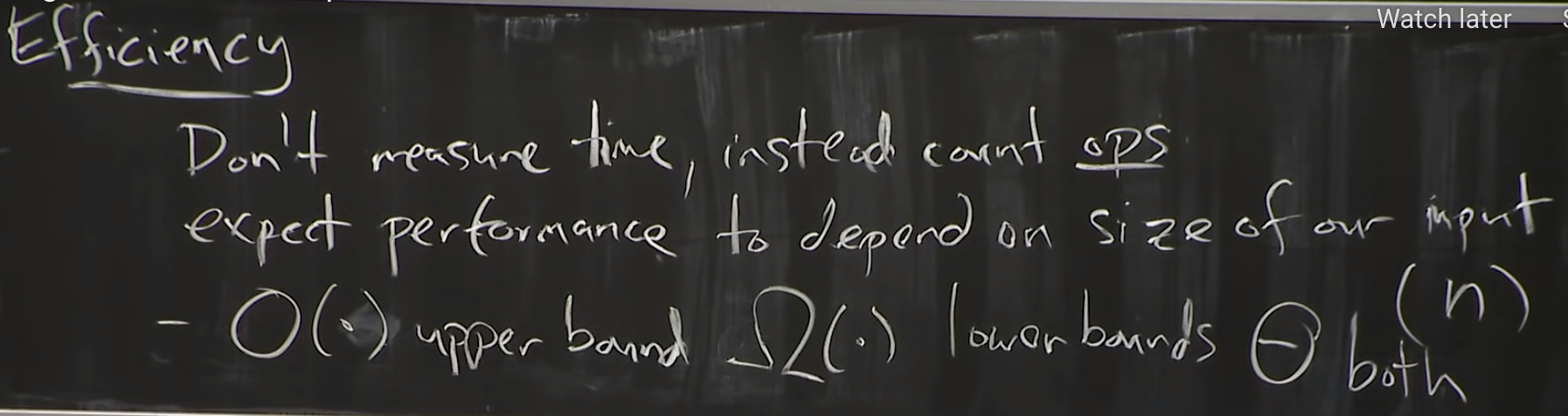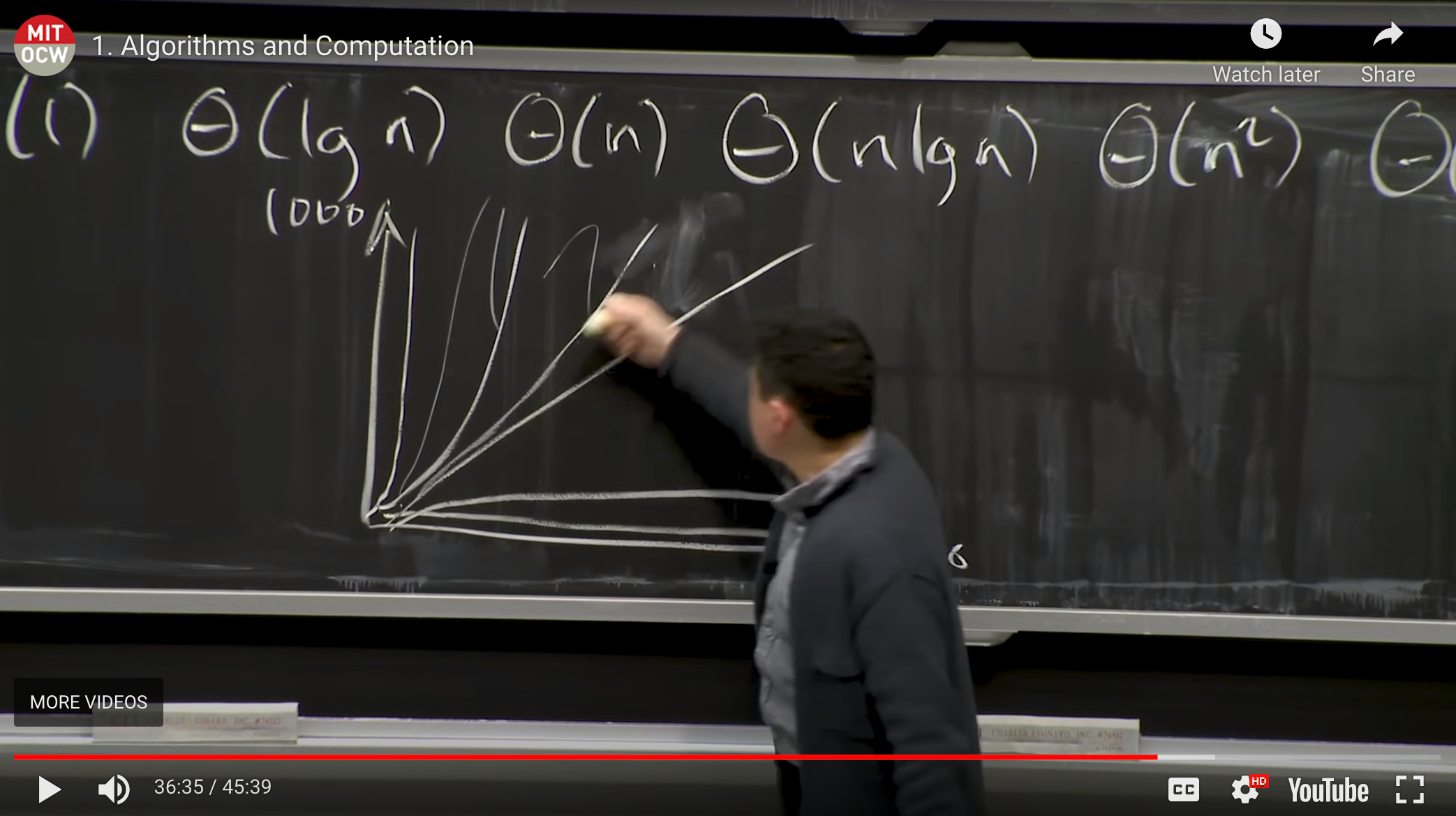UntitledFold the Video

# MIT Algorithms MIT 6006 Lecture 1: Algorithms and computation Summary

The summary of MIT Algorithms MIT 6006 lecture

Goals:

• solve computational problems
• prove correctness
• argue efficiency

A problem

A binary relationship between inputs ans outputs.

Algorithm

A procedure/function that takes inputs and maps it to a correct output, according to thr problem.

• General problems
• arbitrary input sizes

we write a constant size of code that can solve the problem for any arbitrary sized input.

INDUCTIVE PROOF (for correctness)

We need:

• base case
• a hypotheses of something that should be maintained
• inductive step: "I take a smaller size of the problem, use the inductive statement, and urgue it for the larger value of the problem"Efficency

Not only how fast the algorithm runs, but also how fast it runs relative to other algorithms that does the same thing.log(n) is almost as good as constant time. especially as the input size increases

expontential time is the opposite of log in behavior. (really bad)

MODEL OF COMPUTATIONWORD-RAM (RAM): Random access memory (we can randomly access different locations of it in constant time)Memory is just a string of bits of 1s an 0s.

CPU really small: it can hold small amount of information but can act on and change information. It also have instructions to look for info from memory, act on it and send it back.

• we don't have addresses of every bit in memory in our cpu
• a modern computer is addressed in bytes (collections of 8 bits)
• If we want to feed something into the cpu we give it the address of the chunk. (this chunk is a sequence of bits, we call this a WORD; how big of a chunk the CPU can take in from memory and operate on. currently 64 bits.

When the WORD size was 32 bits: we can only address 2**32 addresses in memory. It can only address up to 4GB hard drive.

With 64 bits we can address 20 exabytes addresses (total google data is 10 exabytes)

Operations we can perform on the WORD

• compare 2 WORDS in memory (2 addresses)
• integer arithmetic
• logical operations
• bitwise operations
• read/write from an address in memory

A CPU normally operates on 2 WORDS and produce the 3rd and spits it out. (A CPU operates on constant amout of data)Last Updated:

Summarize & share videos seamlessly